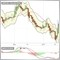233

In one of my indicator, i calculate values...

double a = 0.005403564271234229;

double b = 0.005490378598028686;

MyBuffer1[i] = a/b;   // <---- If i execute this line,  whole  indicator stops functioning completely on chart

MyBuffer1[i] = NormalizeDouble(a,10) / NormalizeDouble(b,10) ;    // <---- This line returns indicator to its normal life

what the problem?22428

2017.04.01 09:50:27.726    testIndi AUDJPY,M1: 0.9841879161437742
That is not the problem, the division works fine. The problem is else where. What does the log say?